# Ordered ring

partially ordered ring

A ring(not necessarily associative) which is a partially ordered group under addition and in which for any elements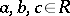the inequalities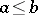and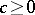imply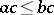and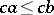. Every ring is an ordered ring for the trivial order. As examples of ordered rings one may take an ordered field; the ring of real functions on a set, wheremeans thatfor all; or a matrix ring over an ordered ring, where, by definition,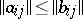if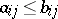for all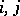. Ifis an ordered ring, then the setis called its positive cone. The positive cone of an ordered ring completely defines the order: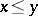if and only if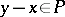. A subsetof a ringcan serve as the positive cone for some order if and only if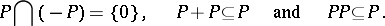The equation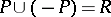is equivalent to the totality of the order (cf. Totally ordered set).

An ordered ring that is totally ordered or lattice-ordered is accordingly called a totally ordered or lattice-ordered ring (cf. also Archimedean ring). Lattice-ordered rings turn out to be distributive lattices, and their additive groups are torsion-free (cf. Lattice-ordered group). Certain questions in the theory of associative rings and, in particular, in the theory of radicals have analogues in associative lattice-ordered rings. The class of rings which allow a lattice-ordered ring structure is not axiomatizable. If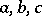are elements of a lattice-ordered ring and, then the following relations hold:Ideals in lattice-ordered rings which are convex subgroups (cf. Convex subgroup) of the additive group are called-ideals. The quotient ring by an-ideal can be made into a lattice-ordered ring in a natural way. The homomorphism theorem holds.

A lattice-ordered ringis called a functional ring or an-ring if it satisfies any of the following equivalent conditions: 1)is isomorphic to a lattice-ordered subring of a direct product of totally ordered rings; 2) for anyone has the implication3) for any subsetofthe set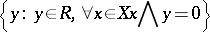is an-ideal; and 4) for any,Condition 4) shows that-rings form a variety of signature. Neither of the equations in this condition is a consequence of the other. Not every-ring can be imbedded in an-ring with a unit element. Ifare elements of an-ring and, then one has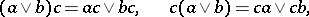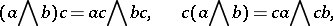as well as the implication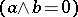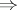.

An order of an ordered ringwith a positive conecan be extended to a total order such thatbecomes a totally ordered ring if and only if for any finite set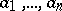inone can choose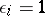or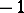such that in the semi-ring generated byand the elements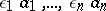the sum of any two non-zero elements is non-zero. With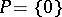one obtains a criterion for the possibility of having a total order on the ring.